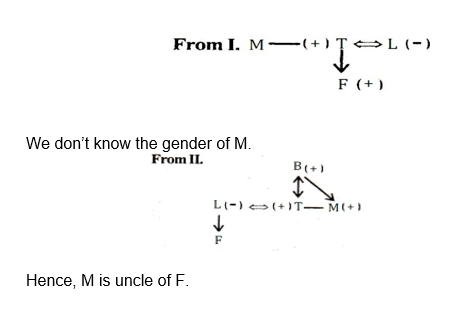# Reasoning Quiz for IBPS SO and CLERK MAINS 2018| 1st January 2019

Reasoning Questions and Reasoning Quiz for IBPS PO Reasoning , IBPS Clerk Reasoning , IBPS RRB Reasoning ,SBI exam Reasoning , and other competitive exams.

Directions (Q1-Q5): Study the following information carefully to answer the given questions.

Eight people K, L, M, N, O, P, Q and R are sitting in a straight line with equal distances between each other, but not necessarily in the same order. Some of them are facing North and some of them are facing south.

K sits at one of the extreme ends of the line. Only three people sit between K and Q. O sits exactly between K and Q.

R sits third to the right of O. L is an immediate neighbor of R and faces south. M sits second to the right of P. M is not an immediate neighbor of Q.

Immediate neighbor of Q face opposite directions(i.e. if one neighbor faces North then the other neighbor faces south and Vice-Versa)

K and N face the same direction as O (i.e. if O faces north then K and N also face North and Vice-Versa). Both the immediate neighbors of O face south. Q faces north.

Q1. How many persons are sitting between P and M?

(a) Two

(b) Three

(c) Four

(d) More than four

(e) One

Q2. Who amongst the following sits third to the right of P?

(a) K

(b) L

(c) Other than those given as options

(c) N

(e) O

Q3. How many people face North as per the given arrangement?

(a) Two

(b) Three

(c) Four

(d) More than four

(e) One

Q4. Four of the following five are alike in a certain way based upon their seating arrangement and so form a group. Which of the following does not belong to the group?

(a) OM

(b) KP

(c) LP

(d) MQ

(e) LQ

Q5. Who amongst the following sits at the extreme end of the row?

(a) L

(b) M

(c) N

(d) O

(e) Other than those given as options

Directions (Q6-Q10): Each of the questions below consists of a question and two statements numbered I and II given below it. You have to decide whether the data provided in the statements are sufficient to answer the question. Read both the statements and give answer.

(a) if the data in statement I alone are sufficient to answer the question, while the data in statement II alone are not sufficient to answer the question.

(b) if the data in statement II alone are sufficient to answer the question, while the data in statement I alone are not sufficient to answer the question.

(c) if the data either in statement I alone or in statement II alone are sufficient to answer the question.

(d) if the data in both the statements I and II together are not sufficient to answer the question.

(e) if the data in both the statements I and II together are necessary to answer the question.

Q6. There are five persons Akansha, Ankita, Puja, Shivangi, and Prakash. Then Ankita is in which direction with respect to Prakash?

1. Akansha is to the west of Shivangi and to the north of Ankita, and Puja is to the south of Prakash.
2. Shivangi is to the north of Akansha and east of Prakash. Ankita is to the west of Puja and south-east of Shivangi.

Q7. What is the angle between the two hands of a clock?

1. One hour ago the angle between the two hands was 75 degrees and the minute hand was ahead of the hour hand.
2. The hour hand is between 9 and 10.

Q8. Among T, F, L, M and B, is M uncle of F?

1. F is son of T, who is brother of M. L is sister-in-law of M.
2. L is mother of F and wife of T, who is son of B, who is father of M and has only two sons and no daughter.

Q9. Between which two letters will be the position of the hour hand of a clock at 9.43 PM?

1. There are consecutive odd-positioned letters of English alphabet on the dial instead of digit.
2. The dial of the clock consists the letter A instead of 1, C instead of 2, E instead of 3, and W instead of 12.

Q10. How many marks did Aman get in English out of 100?

1. When the digits representing Aman’s marks are interchanged. Aman’s marks decreases by 81.
2. Marks obtained by Aman is a two-digit number, whose sum of digits and difference of two digits are same.

# Solutions

Q(1 – 5) :Q1. Ans.(e)

Q2. Ans.(a)

Q3. Ans.(d)

Q4. Ans.(c)

Q5. Ans.(c)

Q6.Ans. (b)

From II.Q7. (a)

Every hour the angle varies by fixed degrees.Q9.Ans. (b)

Q10.Ans. (a)

## WhatsApp Group Join here

Mail us at : ambitiousbaba1@gmail.com